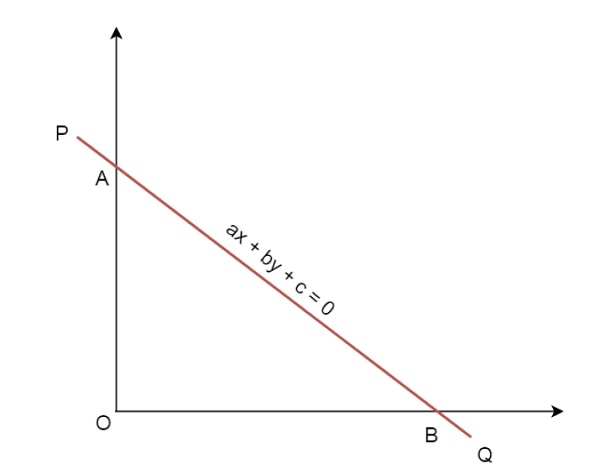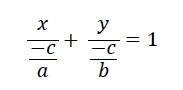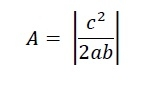# Area of triangle formed by the axes of co-ordinates and a given straight line?

Here we will see how to get the area of a triangle formed by the x and y axis and another straight line. The diagram will be look like below. The equation of the straight line is −

𝑎𝑥+𝑏𝑦+𝑐=0The line is cutting the x-axis at the point B, and cutting the y-axis at the point A. The intercept form will be like below −So the x-intercept is −𝑐∕𝑎 and y-intercept is −𝑐∕𝑏 . So the area of the triangle is## Example

Live Demo

#include<iostream>
#include<cmath>
using namespace std;
double areaTriangle(double a, double b, double c){
return fabs((c*c) / (2*a*b));
}
main() {
double a = -2, b = 4, c = 3;
cout << "Area: " << areaTriangle(a, b, c);
}

## Output

Area: 0.5625